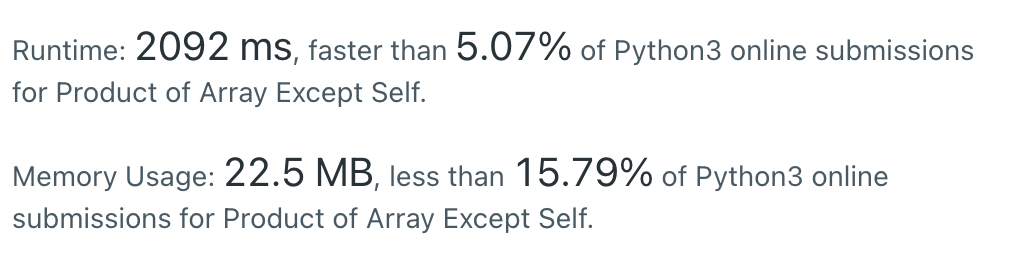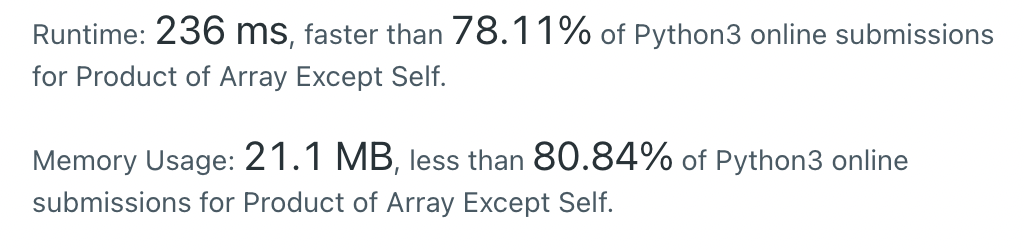#DAY 8
0

## 今日題目：238. Product of Array Except Self

Given an integer array nums, return an array answer such that answer[i] is equal to the product of all the elements of nums except nums[i].

The product of any prefix or suffix of nums is guaranteed to fit in a 32-bit integer.

You must write an algorithm that runs in O(n) time and without using the division operation.

Example 1:
Input: nums = [1,2,3,4]
Output: [24,12,8,6]

### My solution

``````def productExceptSelf(nums):
n = len(nums)
fwd = 
bwd = 
ans = []

for i in range(1,n):
forward = fwd[i-1]*nums[i-1]
fwd.append(forward)
for j in range(1,n):
backward = bwd*nums[n-j]
bwd.insert(0,backward)
for k in range(n):
mid = fwd[k]*bwd[k]
ans.append(mid)
return ans
``````

### Result``````class Solution:
def productExceptSelf(self, nums: List[int]) -> List[int]:
n = len(nums)
fwd = 

for i in range(1,n):
forward = fwd[i-1]*nums[i-1]
fwd.append(forward)
right = 1
for j in range(n-1,-1,-1):
fwd[j] *= right
right *= nums[j]
return fwd
``````